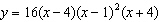Name:    MHF4U - 2.4 Families

Multiple Choice
Identify the choice that best completes the statement or answers the question.

1.

Which of the following is the fully factored form of x3 + 3x2x – 3?
 a. (x + 3)(x2 – 1) c. x2(x + 3) – (x + 3) b. (x – 1)(x + 1)(x + 3) d. (x2 – 1)(x – 3)

2.

What is the maximum number of real distinct roots that a quartic equation can have?
 a. infinitely many c. 2 b. 4 d. none of the above

3.

Which of the following equations has no real solutions?
 a. x2(x2 + 1) = 0 c. 3x3 + 17x2 – 17x – 3 = 0 b. x4 + 20 = 0 d. (x – 1)(x – 2)(x – 3)(x – 4) = 0

4.

A family of quadratic functions has zeros –3 and 5. Which of the following is a member of this family?
 a.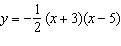c.b.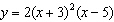d.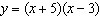5.

Which of the following represents a family of cubic polynomials with zeros –4, 2, and 6?
 a.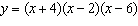c.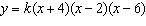b.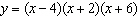d.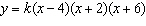6.

Examine the following functions. Which function does not belong to the same family?
 a.c.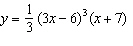b.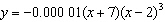d.7.

Examine the graphs of the following polynomial functions. Which graph does not belong to the same family?
 a.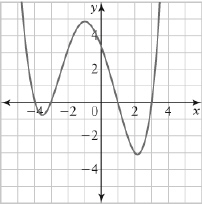c.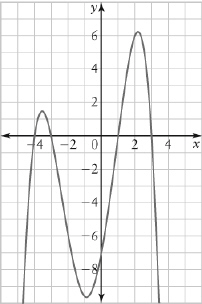b.d.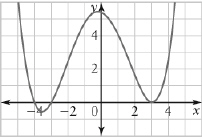8.

What is an equation for the quartic function represented by this graph?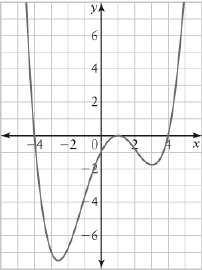a.c.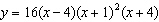b.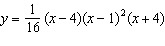d.